5 篇文章 1 订阅

支持向量机

点到平面的距离

平面的一般式方程

w1x1+w2x2+w3x3+b=wTx+b=0

向量的内积

wx=w1x1+w2x2+w3x3

点到平面的距离

!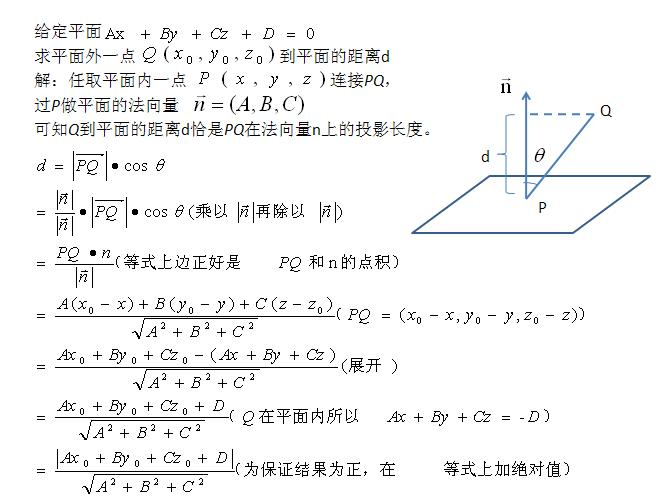最优间隔分类器与支持向量

函数间隔和几何间隔

wTx+b=0

γ(i)=y(i)(wTx(i)+b)

γ(i)=wTx(i)+b

γ=mini=1,...,mγ(i)

r(i)=wTx(i)+bw=γ(i)w

r=mini=1,...,mr(i)

!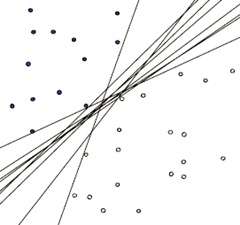如何确定这个超平面

maxr,w,bγws.t.y(i)(wTx(i)+b)γ,i=1,2,...,m

{wTxi+b+1,yi=+1wTxi+b1,yi=1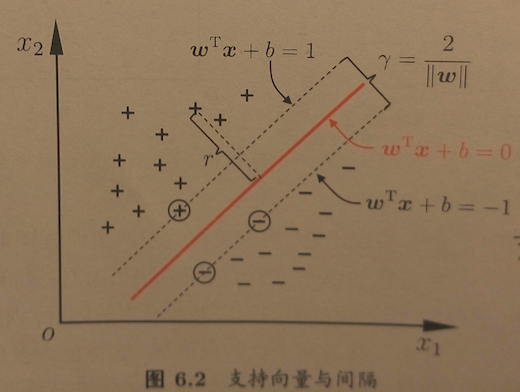γ=2w

最大间隔划分超平面

maxw,b2ws.t.yi(wTxi+b)1,i=1,2,...,m

minw,b12w2s.t.yi(wTxi+b)1,i=1,2,...,m

对偶问题

对偶问题转化

L(w,b,α)=12w2+i=1mαi(1yi(wTxi+b))

θ(w)=maxαi0L(w,b,α)

minw,bθ(w)=minw,bmaxαi0L(w,b,α)=p

maxαi0minw,bL(w,b,α)=d

dp

对偶问题求解

Lw=0w=i=1mαiyixiLb=0i=1mαiyi=0

minw,bL(w,b,α)=12i,j=1mαiαjyiyjxTixji,j=1mαiαjyiyjxTixjbi=1mαiyi+i=1mαi=i=1mαi12i,j=1mαiαjyiyjxTixj

maxαi0i=1mαi12i,j=1mαiαjyiyjxTixjs.t.i=1mαiyi=0,αi0,i=1,2,...,m

f(x)=wTx+b=i=1mαiyixTix+b=i=1mαiyixi,x+b

拉格朗日乘子法和KKT条件

1). 如果目标函数和约束条件都为变量的线性函数, 称该最优化问题为线性规划;
2). 如果目标函数为变量的二次函数, 约束条件为变量的线性函数, 称该最优化问题为二次规划;
3). 如果目标函数或者约束条件为变量的非线性函数, 称该最优化问题为非线性规划.

拉格朗日乘子法–等式约束问题

x=argminxf(x)subject to hi(x)=0,i=1,..,m

x=argmin xL(x,λ)=argmin xf(x)+mi=1λihi(x),

xL(x,λ)=xf(x)+iλixhi(x)=0

L(x,λ)λi=hi(x)=0,

等式约束问题的合理性

minf(x,y)s.t.g(x,y)=c

!Alt text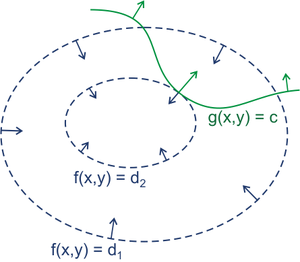[f(x,y)+λ(g(x,y)c)]=0λ0

拉格朗日乘子法–不等式约束问题

x=argminxf(x)

subject to hi(x)=0,i=1,..,msubject to gi(x)0,i=1,..,n

x=argmin xL(x,λ,μ)=argmin xf(x)+mi=1λihi(x)+ni=1μigi(x)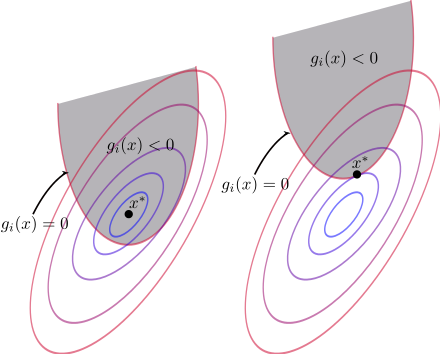μigi(x)=0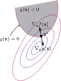{x^*} = \underset{{x}}{\textrm{argmin }} f({x})  + \mu_i g_i({x})
\\0 = \nabla f({x})  + \mu_i \nabla g_i({x})
\\\begin{equation}\mu_i = -\frac{\nabla f({x})}{\nabla g_i({x})}\label{eq:signofmu}\end{equation}

x $x^*$ 点处， f(x) $f(x)$ gi(x) $g_i(x)$ 的梯度方向像相反。所以 μi0 $\mu_{i} \ge 0$

KKT条件

x=argminxf(x)

subject to hi(x)=0,i=1,..,msubject to gi(x)0,i=1,..,n

KKT条件是一个非线性规划问题能有最优化解法的必要和充分条件。KKT条件就是指上面最优化问题中的最小点 x $x^*$ 必须满足下面的条件：
Stationarity:Primalfeasibility:Dualfeasibility:Complementaryslackness:0=f(x)+iλihi(x)+iμigi(x)hi(x)=0,i=1,..,mgi(x)0,i=1,..,nμi0,i=1,..,nμigi(x)=0,i=1,..,m

不等式约束问题的合理性

L(x,μ)=f(x)+k=1qμkgk(x)μk0gk(x)0

μk0gk(x)0}=>μg(x)0

\therefore \begin{equation}\max_{\mu}L(x,\mu)=f(x)\label{a}\end{equation}

\therefore\begin{equation}\min_{x}f(x)=\min_{x}\max_{\mu}L(x,\mu)\label{firsthalf}\end{equation}

maxμminxL(x,μ)=maxμ[minxf(x)+minxμg(x)]=maxμminxf(x)+maxμminxμg(x)=minxf(x)+maxμminxμg(x)

μk0gk(x)0}=>minxμg(x)={0ifμ=0org(x)=0ifμ>0andg(x)<0

maxμminxμg(x)=0

\begin{equation}\therefore \max_{\mu}\min_{x}L(x,\mu)=\min_{x}f(x)+\max_{\mu}\min_{x}\mu{g(x)}=\min_{x}f(x)\label{secondhalf}\end{equation}

minxmaxμL(x,μ)=maxμminxL(x,μ)

L(x,μ)=f(x)+k=1qμkgk(x)μk0gk(x)0
=>
minxmaxμL(x,μ)=maxμminxL(x,μ)=minxf(x)

L(x,μ)=f(x)+k=1qμkgk(x)μk0gk(x)0
=>
minxmaxμL(x,μ)=maxμminxL(x,μ)=minxf(x)=f(x)μkgk(x)=0L(x,μ)x|x=x=0

KKT条件是拉格朗日乘子法的泛化，如果我们把等式约束和不等式约束一并纳入进来则表现为：

L(x,λ,μ)=f(x)+i=1nλihi(x)+k=1qμkgk(x)λi0hi(x)=0μk0gk(x)0

=>
minxmaxμL(x,λ,μ)=maxμminxL(x,λ,μ)=minxf(x)=f(x)μkgk(x)=0L(x,λ,μ)x|x=x=0

L(x,λ,μ)x|x=x=0 $\frac{\partial{L(x,\lambda,\mu)}}{\partial{x}}|_{x=x^*}=0$ 表明 f(x) $f(x)$在极值点 x $x^∗$ 处的梯度是各个 hi(x) $h_i(x^∗)$ gk(x) $g_k(x^∗)$ 梯度的线性组合。

核函数

特征空间的隐式映射

ϕ(x) $\phi \left( x \right)$ 表示将 x $x$ 映射后的特征向量，于是，在特征空间中的划分超平面所对应的模型可表示为

f(x)=wTϕ(x)+b

minw,b12w2s.t.yi(wTϕ(xi)+b)1,i=1,2,...,m

maxαi0i=1mαi12i,j=1mαiαjyiyjϕ(xi)Tϕ(xj)s.t.i=1mαiyi=0,αi0,i=1,2,...,m

ϕ(xi)Tϕ(xj) $\phi \left( x_i \right)^T{\phi \left( x_j \right)}$ 可以有两种方法
1、先找到这种映射，然后将输入空间中的样本映射到新的空间中，最后在新空间中去求内积
2、或者是找到某种方法，它不需要显式的将输入空间中的样本映射到新的空间中，而能够在输入空间中直接计算出内积，这就是传说中的核函数方法

k(xi,xj)=ϕ(xi),ϕ(xj)=ϕ(xi)Tϕ(xj)

maxαi0i=1mαi12i,j=1mαiαjyiyjk(xi,xj)s.t.i=1mαiyi=0,αi0,i=1,2,...,m

f(x)=wTϕ(x)+b=i=1mαiyiϕ(xi)Tϕ(x)+b=i=1mαiyik(xi,x)+b

核函数有效性判定

k(x,z)=(xTz)2=(i=1nxizi)j=1nxjzj=i=1nj=1n(xixj)(zizj)=ϕ(x)Tϕ(z)

ϕ(x)=[x1x1x1x2x1x3x2x1x2x2x2x3x3x1x3x2x3x3]T

k(x,z)=(xTz+c)2=(i=1nxizi+c)j=1nxjzj+c=i=1nj=1n(xixj)(zizj)+(i=1n(2cxi)(2czi))+c2

ϕ(x)=[x1x1x1x2x1x3x2x1x2x2x2x3x3x1x3x2x3x32cx12cx22cx3c]T

半正定矩阵

1.实对称矩阵 A$A$ 的不同特征值对应的特征向量是正交的。
2.实对称矩阵 A $A$ 的特征值都是实数，特征向量都是实向量。
3.n$n$ 阶实对称矩阵 A $A$ 必可对角化，且相似对角阵上的元素即为矩阵本身特征值。

有效地核函数定义

Kij=K(x(i),x(j))=ϕ(xi)Tϕ(xj)=ϕ(xj)Tϕ(xi)=K(x(j),x(i))=Kji

zTKz=i=1nj=1n(ziKijzj)=i=1nj=1n(ziϕ